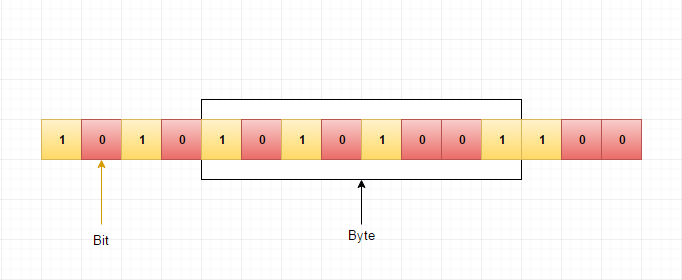# How data is stored in computer memory

As we all know, computer is a electronic device where data is stored in the form of electronic signals.

Bit is the basic unit of memory. At a time, it can be either on or off. Generally, bits are represented using electrical voltage.

Voltage presense indicates that the bit is in ON state. Voltage absense indicates that the bit is in OFF state.

Here, OFF state is considered as 0. ON state is considered as 1.

Computer memory is the collection of several bits. Group of 8 bits are called byte.

#### Pictorial Explanation## Useful Resources

https://en.wikipedia.org/wiki/Memory_cell_(binary)
https://en.wikipedia.org/wiki/Bit
https://en.wikipedia.org/wiki/Byte